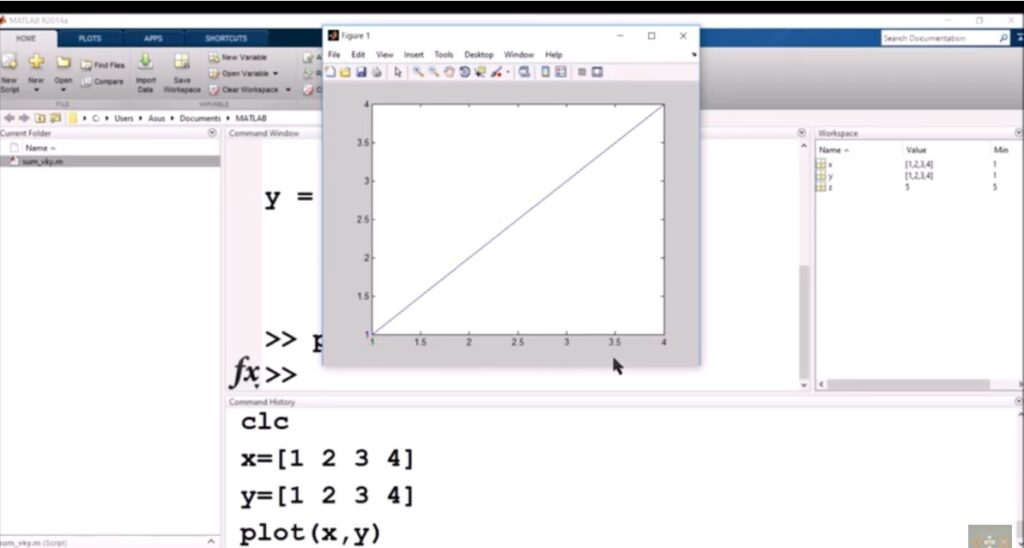## MATLAB A POWERFUL TOOL FOR THE ENGINEERS

MATLAB® is a programming platform designed specifically for engineers and scientists. The heart of MATLAB is the MATLAB language, a matrix-based language allowing the most natural expression of computational mathematics. MATLAB combines a desktop environment tuned for iterative analysis and design processes with a programming language that expresses matrix and array mathematics directly. What can you …